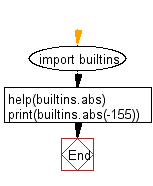﻿ Python: Documentation of a built-in-function - w3resource# Python: Documentation of a built-in-function

## Python Class ( Basic ): Exercise-4 with Solution

Write a python program which import the abs() function using the builtins module, display the documentation of abs() function and find the absolute value of -155.

Sample Solution:

Python Code:

``````import builtins
help(builtins.abs)
print(builtins.abs(-155))
```
```

Sample Output:

```Help on built-in function abs in module builtins:

abs(x, /)
Return the absolute value of the argument.

155
```

Flowchart:## Visualize Python code execution:

The following tool visualize what the computer is doing step-by-step as it executes the said program:

Python Code Editor:

What is the difficulty level of this exercise?

Test your Programming skills with w3resource's quiz.

﻿

## Python: Tips of the Day

The Zip() Function:

```>>> students = ('John', 'Mary', 'Mike')
>>> ages = (15, 17, 16)
>>> scores = (90, 88, 82, 17, 14)
>>> for student, age, score in zip(students, ages, scores):
...     print(f'{student}, age: {age}, score: {score}')
...
John, age: 15, score: 90
Mary, age: 17, score: 88
Mike, age: 16, score: 82
>>> zipped = zip(students, ages, scores)
>>> a, b, c = zip(*zipped)
>>> print(b)
(15, 17, 16)
```